# Math Practice Topic: Angle Measurements 2

 Description: Solve problems involving angle relationships: vertical angles, parallel lines cut by a transversal, and angles around a point. Adaptive Learning Progression: The figures involved become more complex. Start using MathScore for free

## Sample Levels (out of 4)

Solve for the missing angle(s).
Figures are not drawn to scale.

1.
In the figure below, two lines intersect to form ∠A,
B, ∠C, and ∠D.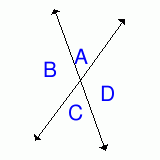If m∠C = 55°, find the missing angles.
m∠A = °
m∠B = °
m∠D = °
2.
In the figure below, two lines intersect to form ∠A,
B, ∠C, and ∠D.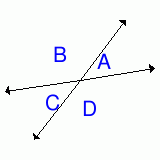If m∠B = 136°, find the missing angles.
m∠A = °
m∠C = °
m∠D = °

Solve for the missing angle(s).
Figures are not drawn to scale.

1.
In the figure below, lines g and h are parallel, and line m is a transversal.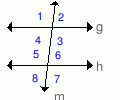If m∠2 = 85°, what is m∠7?      °
2.
In the figure below, lines g and h are parallel, and line m is a transversal.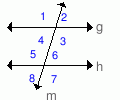If m∠8 = 74°, what is m∠4?      °
3.
In the figure below, lines g and h are parallel, and line m is a transversal.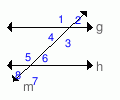If m∠1 = 126°, what is m∠8?      °
4.
In the figure below, lines g and h are parallel, and line m is a transversal.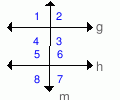If m∠4 = 94°, what is m∠8?      °

Solve for the missing angle(s).
Figures are not drawn to scale.

1.If m∠C = 35° and m∠A + m∠B + m∠E = 240°, what is m∠D?   °
2.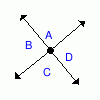If all 4 angles are equal, what is the measure of one angle?   °
3.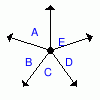If all 5 angles are equal, what is the measure of one angle?   °

Solve for the missing angle(s).
Figures are not drawn to scale.

1.
In the figure below, lines g and h are parallel, and line m is a transversal.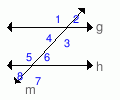If m∠8 = 42°, find the missing angles.
m∠1 = °
m∠3 = °
m∠6 = °
2.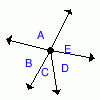If m∠B = m∠E and m∠A + m∠C + m∠D = 212°, what is m∠E?   °
3.
In the figure below, three lines intersect to form a triangle with interior angles W, F, and D.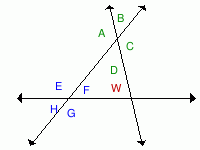If m∠W = 80° and m∠F = 60°, what is m∠C?   °